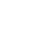Live chatTable of Contents

## 2.734

From the information on the stocks of the XYZ Company, the following values were recorded:

1. XYZ's current annual dividend = \$0.95
2. XYZ's beta (ß)  = 1.75
3. Industry P/E = 24.6
4. XYZ's 3-year dividend growth rate (g) = 8.52%
5. XYZ's EPS. = \$5.32

Having the information above, the use of the CAPM (Capital Asset Pricing Model) to calculate XYZ’s rate of return (ks) would give;

Ks = KRF + b(market Risk Premium)

Ks =2.734 + 1.75(7.5)

= 15.86%

Using the CGM method of calculations, and calling the theoretical price (Po), the formula to used to calculate the stock price is.

The annual growth rate being 8.2%, and the Current Annual Dividend (D0) being \$0.8, then expected stock dividend (D1) would be calculated as the annual growth rate multiplied by D0

\$0.95 * 1.0852 = \$1.0309

Then

= \$14.045

After using the web resources that are relevant, I have decided on the current XYZ’s stick quote to be 77.64.

On comparing the two values of Po and P it is very evident that the two values are different. The difference is

77.63-14.045 = 63.585

These kinds of differences can be caused by many different factors, amongst them are;

Stay ConnectedThere is an assumption made in calculating the rate of return. This is the market risk premium that is the difference between the required rate of return in the market and the rate of return that is risk free. This premium has been assumed at 7.5%. In most cases the market risk premium shifts every day, and when this happens, it would affect the company’s required rate of return. This will obviously affect the calculated stock price as it forms part of the equation.

The growth rate of the XYZ Company is likely to be higher than the one that have been used here. With a higher rate than the 9.15% in the calculations, this would result into a different price per share, a higher one obviously, of 16.1%.

During the past month, the price per share of the XYZ Company has been increasing. This must have encouraged speculators and impulse buyers to invest heavily on the stocks belonging to XYZ. This also could have made the share prices to increase.

Assuming that the market risk premium rose up from 7.5% to 10% and that the reason is solely due to greater risks in the market. This hence means the risk free interest rate and the beta value of the stocks remained the same. The new price would then be

Ks = 2.734 + 1.75(10)

= 20.234%

P0 = \$8.8

This change is evidence of the increase in the risks. We see that P0 has reduced from 14.047 to 8.8 dollars. This higher risk rate will result into a lower price per share because higher risk premium is translated into higher risks. Investors being always profit oriented, they would want a higher return on their investments so as to cover up for this upward shift on risks.

In the recalculation of XYZ’s stock price using the P/E ratio model and making use of the much required information from the XYZ Stock Information file.

Limited time Offer

0
0
days
:
0
0
hours
:
0
0
minutes
:
0
0
seconds
Get 19% OFF

XYZ EPS = \$5.32

Industry P/E Ratio = 24.6

Stock price = (EPS) (P/E Ratio)

Therefore    5.32*24.6 = \$130.87

This stock price is different from that which was obtained in the initial CGM (Constant Growth Model) because this ratio used above lays its basis on. These include the company’s expectation on future dividends and its ability to grow exponentially.  Therefore it is clear that while the Constant Growth Model is based on past activities, the PE ratio model bases itself on what is expected in the future.

0

Preparing Orders

0

Active Writers

0

Support Agents

Limited offerget 15% off your 1st order with code first15